### 4.2.2.2 Response Surface Method

The response surface method (RSM) has been introduced by Box and Wilson  and approximates the original problem (4.1) by a surrogate problem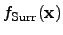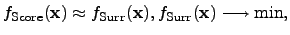(4.19)

where the surrogate function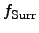estimates the original function. The surrogate function is often expressed by an analytical function inand sample points of the original function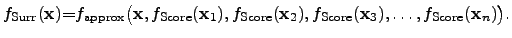(4.20)

A common method is to use an n-dimensional polynomial function, where the evaluated parameter sets provide base points for interpolation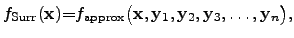(4.21)

with the values of the evaluated score functions denoted as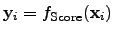. This RSM method can be applied if the evaluation of the original function is rather expensive in terms of the computational effort as well as with respect to the computational resources needed for the evaluation of the original problem.

The main goal of this method is to describe the original function with as few sample points as possible to minimize the computational effort for evaluating the original function and to speed up the optimization by optimizing a surrogate function.

As a drawback, the more sample points are required for the optimization problem the more expensive is the optimization of the surrogate problem because each new sample point demands a new calculation of the coefficients of the surrogate problem which entails a high computational burden and a huge memory consumption. Nevertheless, if the computational effort for the evaluation of the original problem is huge and the numerical evaluation is very time consuming, the huge resource demands for the surrogate problem is still economically justifiable and reasonable in terms of the computational and optimization time.

Despite of these drawbacks, this method is commonly used in a wide range of applications [238,239,240,241] where large numbers of numerical evaluations of a certain original problem are economically not reasonable within an optimization run.

Stefan Holzer 2007-11-19